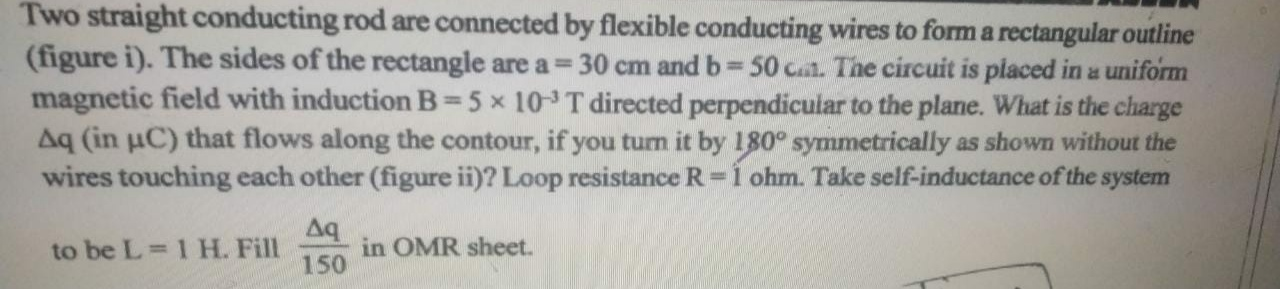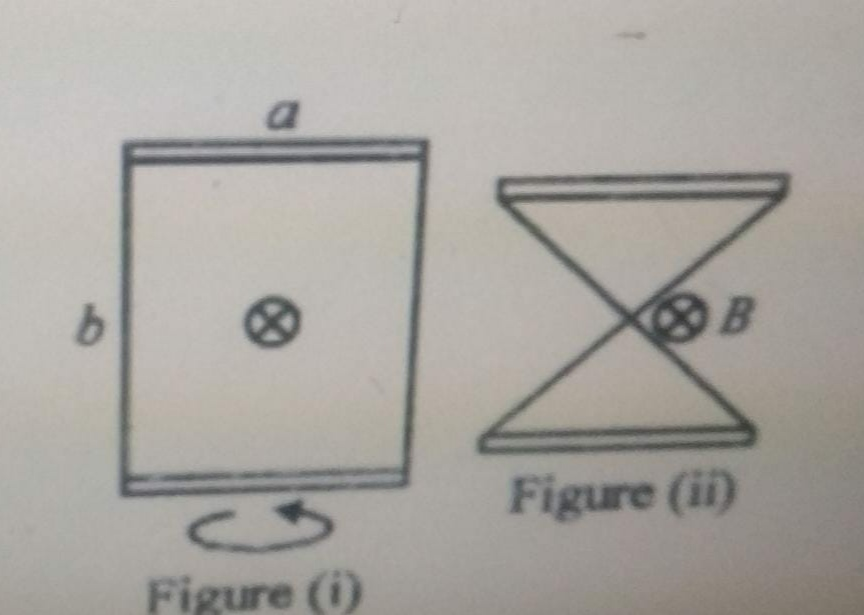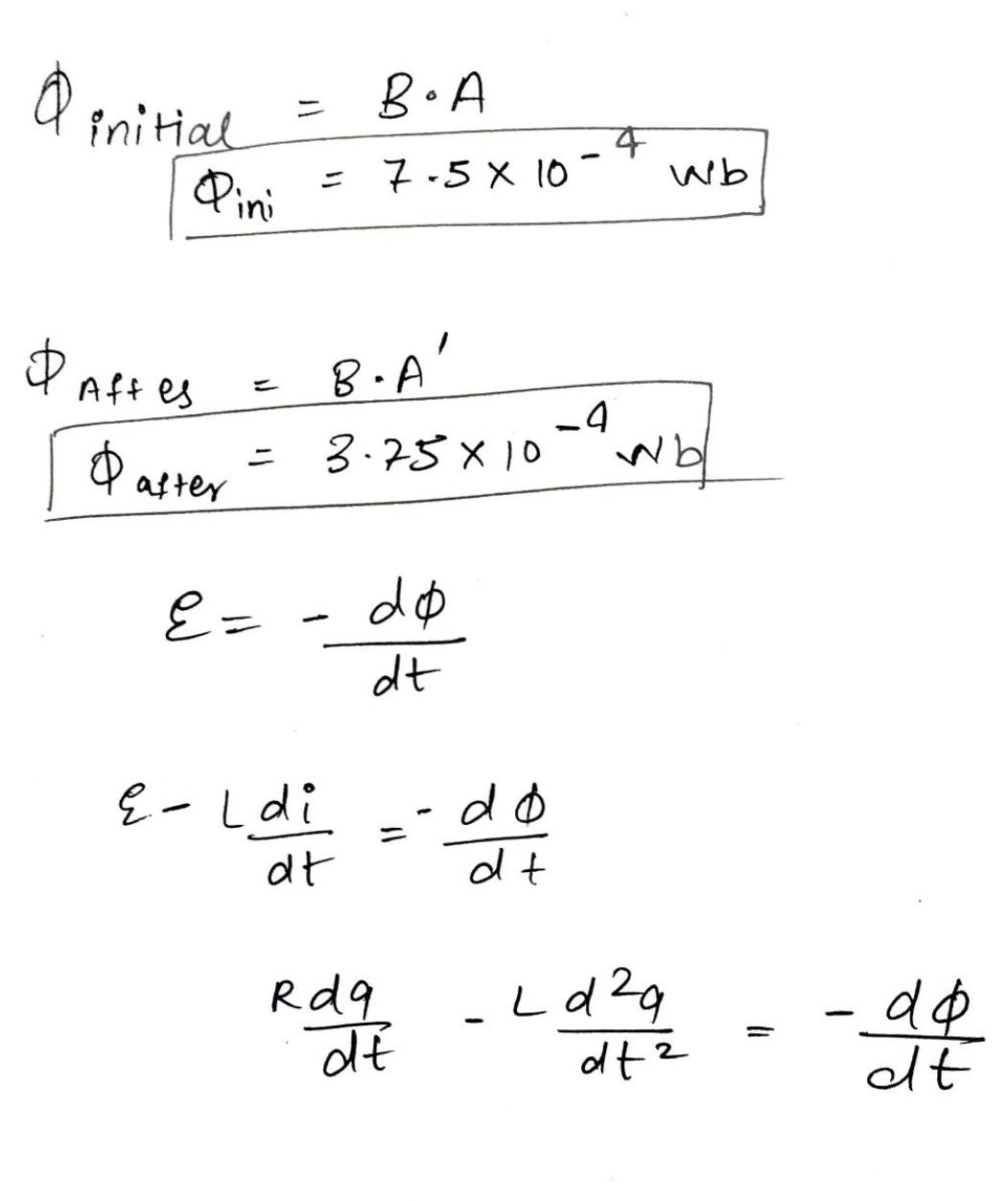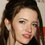# Magnetism DoubtHere is my attempt. Ask anything. I am not sure. :)Note by Talulah Riley
10 months ago

This discussion board is a place to discuss our Daily Challenges and the math and science related to those challenges. Explanations are more than just a solution — they should explain the steps and thinking strategies that you used to obtain the solution. Comments should further the discussion of math and science.

When posting on Brilliant:

• Use the emojis to react to an explanation, whether you're congratulating a job well done , or just really confused .
• Ask specific questions about the challenge or the steps in somebody's explanation. Well-posed questions can add a lot to the discussion, but posting "I don't understand!" doesn't help anyone.
• Try to contribute something new to the discussion, whether it is an extension, generalization or other idea related to the challenge.

MarkdownAppears as
*italics* or _italics_ italics
**bold** or __bold__ bold
- bulleted- list
• bulleted
• list
1. numbered2. list
1. numbered
2. list
Note: you must add a full line of space before and after lists for them to show up correctly
paragraph 1paragraph 2

paragraph 1

paragraph 2

[example link](https://brilliant.org)example link
> This is a quote
This is a quote
    # I indented these lines
# 4 spaces, and now they show
# up as a code block.

print "hello world"
# I indented these lines
# 4 spaces, and now they show
# up as a code block.

print "hello world"
MathAppears as
Remember to wrap math in $$ ... $$ or $ ... $ to ensure proper formatting.
2 \times 3 $2 \times 3$
2^{34} $2^{34}$
a_{i-1} $a_{i-1}$
\frac{2}{3} $\frac{2}{3}$
\sqrt{2} $\sqrt{2}$
\sum_{i=1}^3 $\sum_{i=1}^3$
\sin \theta $\sin \theta$
\boxed{123} $\boxed{123}$

Sort by:

The final flux you calculated is not correct. But you are not on the wrong track. I will share my solution after some time. This is a very nice conceptual question. I do not know if my answer is correct though. You should think about this problem too.

- 10 months ago

In my opinion your answer is correct. Because my friend was practicing physics for JEE exam.

And in this exam answer is probably a good number like (1, 5,10,12,) it is not like answer will come 3.873,4.273,9.282.

- 10 months ago

@Karan Chatrath But How my $\phi_{final}$ is incorrect?

- 10 months ago

@Karan Chatrath sorry for the delay.posting attempt till 11:30

- 10 months ago

@Karan Chatrath I have uploaded my attempt above.

- 10 months ago

@Steven Chase Hello,in case you want to contribute something. You are welcome.

- 10 months ago

Say the area vector points into the plane, in the direction of the magnetic field. Consider the initial flux:

$\Phi_{i} = \vec{B} \cdot \vec{A} = Bab$

Now consider the geometry in the final configuration. In the final configuration, had the area of the loop is turned by 180 degrees. The area of one half is:

$\vec{A}_1 = \frac{ab}{4} \hat{k}$ $\vec{A}_2 = -\frac{ab}{4} \hat{k}$

Now, the final flux is:

$\Phi_{f} = \vec{B} \cdot \vec{A}_1 + \vec{B} \cdot \vec{A}_2= 0$

Now, let us apply KVL in the loop. the equation would be:

$\lvert \frac{d\Phi}{dt}\rvert = L\frac{dI}{dt} + IR$

Now, consider the system to move from it's initial state to its final state in a time $\Delta t$. The initial and final current must be zero. So KVL can also be written as:

$\lvert \frac{\Delta \Phi}{\Delta t}\rvert = L\frac{\Delta I}{\Delta t} + R\frac{\Delta Q}{\Delta t}$

Now, between initial and final states, $\Delta I=0$, so therefore:

$\lvert \frac{\Delta \Phi}{\Delta t}\rvert = 0+ R\frac{\Delta Q}{\Delta t}$

$\implies \Delta \Phi = R \Delta q$

- 10 months ago

@Karan Chatrath in the 4th step, there are two $=$.

- 10 months ago

Edited solution.

- 10 months ago

@Karan Chatrath the total final flux will cancel out isn't. Because area vectors are opposite. I am bit confused about that?

- 10 months ago

Yes, that is what will happen, according to me.

- 10 months ago

@Karan Chatrath Can be both area vectors in same. How you are deciding direction?.

- 10 months ago

I do not understand your question.

- 10 months ago

@Karan Chatrath the question is “How you know that the direction of flux through both the triangles are opposite? ”

- 10 months ago

This is now I have understood the problem. One of the conducting rods is turned by 180 degrees to achieve the final configuration. So a part of the area is still pointing in its initial direction, but the other half turns by 180 degrees thereby making it oppositely directed.

- 10 months ago

@Karan Chatrath Oh wow! I was also thinking the little bit same. Thanks for clarification.

- 10 months ago

@Karan Chatrath How do you get 5 only?

- 10 months ago

@Karan Chatrath I always consider SI units in Physics

- 10 months ago

@Karan Chatrath wait one last doubt on this question.
I didn't understand 6th to 7th step.? Why the initial and final currents are 0?

- 10 months ago

Because the emf is only induced as long as the loop is turning. Once the loop stops turning, there is no induced emf because flux is no longer changing, so the current decays to zero.

- 10 months ago

@Karan Chatrath Oh yes.Thank you so much.

- 10 months ago

@Karan Chatrath I didn't understand this Problem .
I want to know how to find compression and extension.HELP ME WHENEVER YOU WILL BE FREE.

- 10 months ago

I think I will post a note or a problem related to this problem, later.

- 10 months ago

@Karan Chatrath Thank. I am waiting. By the way my SHM is bit weak. After solving many many problems , I always gets stuck in a new problem.

- 10 months ago

The problem with SHM problems is that while preparing for JEE type exams, people use a lot of shortcut methods to solve them. These shortcuts are different for different types of questions and are not very easy to understand. Treat any SHM problem as a regular mechanics problem.

- 10 months ago

@Karan Chatrath Exactly. You are absolutely correct.
There are many shortcuts in physics which we use in JEE and when we post those problems in Brilliant., no one use that short cut , You and Steven sir always solve those questions from basic method like a physicist.
By the way do you have given JEE exam?

- 10 months ago

Yes, I appeared for that exam but I did not clear it. I did not do well.

- 10 months ago

@Karan Chatrath Here is the answer to your all questions respectively. 1)Yes
2)No
3)Would you like to see my solution?

- 9 months, 3 weeks ago

I would like to see your approach. No need to post the entire solution.

- 9 months, 3 weeks ago

@Karan Chatrath my approach is only use of kinematics and simple harmonic motion.

- 9 months, 3 weeks ago

I want you to describe your approach and not give me a generic answer. Describe your steps.

1. I analysed the time taken for the two dumbbells to first come into contact.
2. At that instant, I applied momentum and energy conservation to calculate the final velocities of the two masses that collide, after collision. The other two masses' velocities remain unchanged.
3. I use the positions and velocities of all the masses as initial conditions to solve the differential equations of motion after collision.

- 9 months, 3 weeks ago

@Karan Chatrath here are some steps which i hae followed.
1)Momentum conservation.
2)Reduced mass system.
3)Simple harmonic
4)Kinematics.

- 9 months, 3 weeks ago

You are not elaborating. Nevermind. I have changed my mind. You may share your solution.

- 9 months, 3 weeks ago

@Karan Chatrath Sorry I don't think I can elaborate more. Because there is nothing in the question which needs to be elaborated. And my english is also not so good. I will upload the solution on 4:00PM today. Bye

- 9 months, 3 weeks ago

@Talulah Riley Hey are you there?

- 9 months ago

@Karan Chatrath I am getting answer as $5×10^{-6}$

- 10 months ago

Read the question. The answered is required in $\mu C$.

- 10 months ago

@Karan Chatrath Sorry, I understand everything. $THANKS$

- 10 months ago